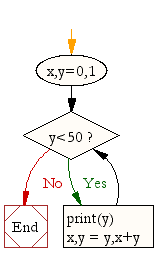## ~ OOP,Classes,Python,Tkinter ...recreating PONG

### Code and Challenge

Copy and paste the code below. Run it! Read the task and see if you can do it before moving on to the next challenge

```"""

This additional code ends the game for you - if the ball hits the bottom - all movement stops!
See if you can use your knowledge of TKINTER to add a proper "Game Over" Label to appear on the screen, when the game is over!
(before looking ahead to the next challenge which contains the solution)
"""

from tkinter import *
import random
import time

class Ball:
def __init__(self,canvas,bat,color):
self.canvas=canvas
self.bat=bat
self.id=canvas.create_oval(30,30,50,50,fill=color)
self.canvas.move(self.id,100,200)
starting_position=[-3,-2,-1,1,2,3]
random.shuffle(starting_position)
self.x = starting_position
self.y = -3
self.canvas_height=self.canvas.winfo_height()
self.canvas_width=self.canvas.winfo_width()
#Add a hit_bottom object variable here..
self.hit_bottom=False #...note we change the main loop at the bottom to include an if function that utilises this hit_bottom object variable

def hit_bat(self,pos):
bat_pos=self.canvas.coords(self.bat.id)
if pos >=bat_pos and pos <=bat_pos:
if pos>=bat_pos and pos <= bat_pos:
return True
return False

def draw(self):
self.canvas.move(self.id,self.x,self.y)
pos=self.canvas.coords(self.id)
if pos <=0:
self.y=6
#we make a change here as well -alter the if statement to see if the ball has hit the bottom (equal or greater than canvas height), if so hit_bottom =True (as there is no more need to bounce the ball if the game is over!)
if pos >=self.canvas_height:
self.hit_bottom = True

if self.hit_bat(pos) ==True:
self.y=-6
if pos <=0:
self.x=6
if pos>=self.canvas_width:
self.x=-6

class Pongbat():
def __init__(self,canvas,color):
self.canvas=canvas
self.id=canvas.create_rectangle(0,0,100,10,fill=color)
self.canvas.move(self.id,200,300)
self.x=0
self.canvas_width=self.canvas.winfo_width()
self.canvas.bind_all('',self.left_turn)
self.canvas.bind_all('',self.right_turn)

def draw(self):
self.canvas.move(self.id,self.x,0)
pos=self.canvas.coords(self.id)
if pos<=0:
self.x=0
if pos>=self.canvas_width:
self.x=0

def left_turn(self,evt):
self.x=-10

def right_turn(self,evt):
self.x=10

def main():
tk=Tk()
tk.title("My 21st Century Pong Game")
tk.resizable(0,0)
tk.wm_attributes("-topmost",1)
canvas=Canvas(tk,bg="white",width=500,height=400,bd=0,highlightthickness=0)
canvas.pack()
tk.update()

bat1=Pongbat(canvas,'red')
ball1=Ball(canvas,bat1, 'green')

while 1:
if ball1.hit_bottom ==False: #this creates a condition - inside the loop it continues to check to see if the ball has hit (or not) the bottom of the screen
tk.update()
ball1.draw()
bat1.draw()
time.sleep(0.02)
main()

#note - an ERROR may be caused as Python is complaining about breaking out of the while loop when the window is closed - you can ignore that for now (or a fix is provied in the solutions section for those who are interested"""

```

Systems Life Cycle (in a nutshell): Analyse - Design - Create - Test - Evaluate. Designing something or writing out some pseudocode before you actually write code is always a good idea! Get in to the habit of doing so! You can draw your flowchart here and screenshot it.

A sample flow chart (design) for this particular challenge could look like:Each challenge section below provides an online drawing tool where you can dynamically create flowcharts. Screenshot them into your presentation for submission.